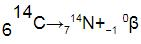Courses

# Radioactivity - Nuclei

## 5 Questions MCQ Test Physics Class 12 | Radioactivity - Nuclei

Description
This mock test of Radioactivity - Nuclei for JEE helps you for every JEE entrance exam. This contains 5 Multiple Choice Questions for JEE Radioactivity - Nuclei (mcq) to study with solutions a complete question bank. The solved questions answers in this Radioactivity - Nuclei quiz give you a good mix of easy questions and tough questions. JEE students definitely take this Radioactivity - Nuclei exercise for a better result in the exam. You can find other Radioactivity - Nuclei extra questions, long questions & short questions for JEE on EduRev as well by searching above.
QUESTION: 1

### The control rods in a nuclear reactor

Solution:

Control rods are used in nuclear reactors to control the fission rate of uranium and plutonium. They are composed of chemical elements such as boron, silver, indium and cadmium that are capable of absorbing many neutrons without themselves fissioning.

QUESTION: 2

### A sample of radioactive material contains 1018 atoms. The half life of the material is 2 days, then the activity of the sample is​

Solution:

To find activity of the sample --->which is the rate of disintegration.

Since radioactivity comes under 1o kinetics.

[R]=k[A]     [A]-->amount of initial sample 1018 atoms

Given,

Half-life=2days

K=0.693/2x24x60x60 sec

R=(0.693/2x24x60x60)x1018

R≈3.5x1012 Bq

QUESTION: 3

### γ (Gamma) rays are​

Solution:

A gamma ray, or gamma radiation, is a penetrating form of electromagnetic radiation arising from the radioactive decay of atomic nuclei. It consists of the shortest wavelength electromagnetic waves and so imparts the highest photon energy.

QUESTION: 4

When a radioactive nucleus emits a β – particle, the mass number of the atom

Solution:

A beta particle forms when a neutron changes the mass number stays the same.
Example
Carbon-14 decays into nitrogen-14 by emitting a beta particle. This is the balanced equation for the reaction:Notice that:

• the mass number of the nucleus stays the same, 14
• the atomic number of the nucleus increases by 1, from 6 to 7
• the numbers at the top and bottom give the same totals on both sides, as (7 - 1) = 6

Nitrogen nuclei have 7 protons, so their nuclear charge is +7. They have one more proton than carbon nuclei, which have 6 protons and so a nuclear charge of +6.

QUESTION: 5

The purpose of moderator in a nuclear reactor is​

Solution:

In nuclear engineering, a neutron moderator is a medium that reduces the speed of fast neutrons, ideally without capturing any, leaving them as thermal neutrons with only minimal (thermal) kinetic energy.